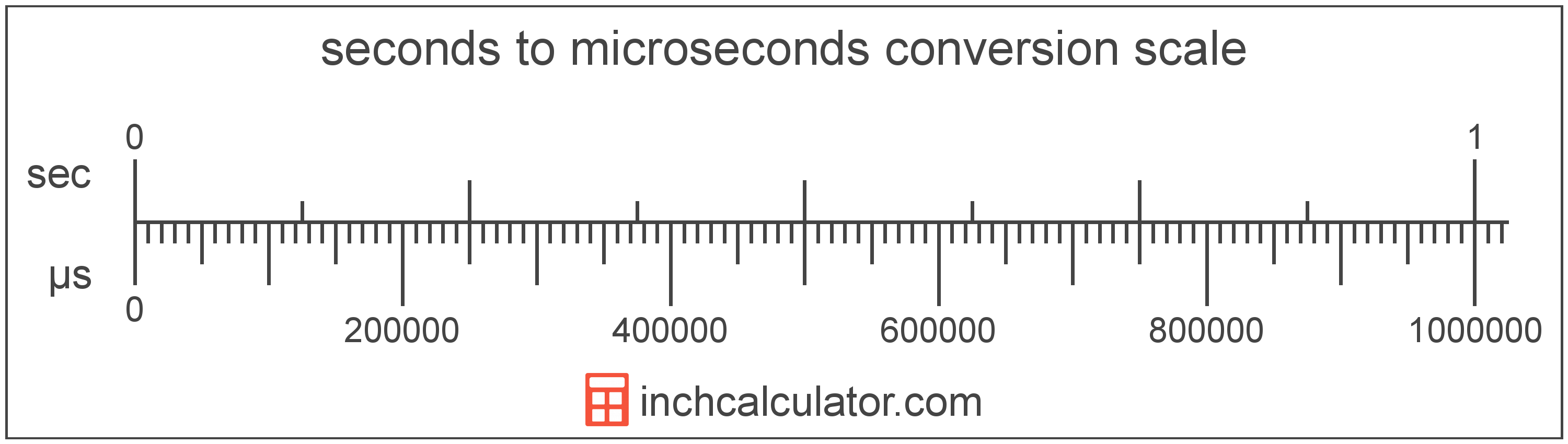# Microseconds to Seconds Converter

Enter the time in microseconds below to get the value converted to seconds.

Results in Seconds:1 µs = 1.0E-6 s
Hint: use a scientific notation calculator to convert E notation to decimal

Do you want to convert seconds to microseconds?

## How to Convert Microseconds to Seconds

To convert a measurement in microseconds to a measurement in seconds, divide the time by the following conversion ratio: 1,000,000 microseconds/second.

Since one second is equal to 1,000,000 microseconds, you can use this simple formula to convert:

seconds = microseconds ÷ 1,000,000

The time in seconds is equal to the time in microseconds divided by 1,000,000.

For example, here's how to convert 5,000,000 microseconds to seconds using the formula above.
seconds = (5,000,000 µs ÷ 1,000,000) = 5 s## What is a Microsecond?

One microsecond is equal to 1/1,000 of a millisecond or 1,000 nanoseconds.

The microsecond is a multiple of the second, which is the SI base unit for time. In the metric system, "micro" is the prefix for millionths, or 10-6. Microseconds can be abbreviated as µs; for example, 1 microsecond can be written as 1 µs.

## What is a Second?

The second has historically been defined as 1/60 of a minute or 1/86,400 of a day. According to the SI definition, the second is the duration of 9,192,631,770 oscillations of the frequency of light emitted from the atomic transition between the two hyperfine levels of the unperturbed ground state of the caesium 133 atom.

The second is the SI base unit for time in the metric system. Seconds can be abbreviated as s, and are also sometimes abbreviated as sec. For example, 1 second can be written as 1 s or 1 sec.

## Microsecond to Second Conversion Table

Table showing various microsecond measurements converted to seconds.
Microseconds Seconds
1 µs 0.000001 s
2 µs 0.000002 s
3 µs 0.000003 s
4 µs 0.000004 s
5 µs 0.000005 s
6 µs 0.000006 s
7 µs 0.000007 s
8 µs 0.000008 s
9 µs 0.000009 s
10 µs 0.00001 s
100 µs 0.0001 s
1,000 µs 0.001 s
10,000 µs 0.01 s
100,000 µs 0.1 s
1,000,000 µs 1 s

## References

1. International Bureau of Weights and Measures, The International System of Units, 9th Edition, 2019, https://www.bipm.org/documents/20126/41483022/SI-Brochure-9-EN.pdf